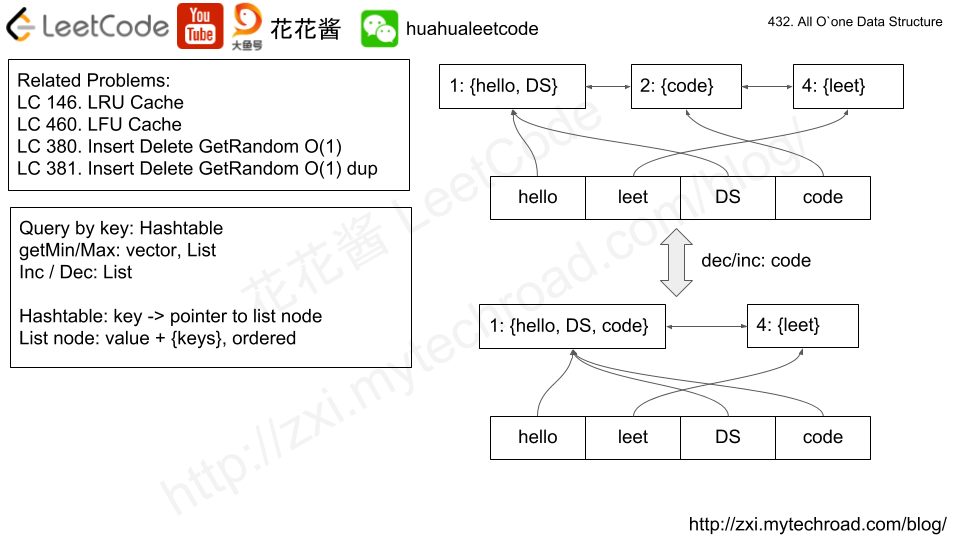# Posts tagged as “design”

Implement a SnapshotArray that supports the following interface:

• SnapshotArray(int length) initializes an array-like data structure with the given length.  Initially, each element equals 0.
• void set(index, val) sets the element at the given index to be equal to val.
• int snap() takes a snapshot of the array and returns the snap_id: the total number of times we called snap() minus 1.
• int get(index, snap_id) returns the value at the given index, at the time we took the snapshot with the given snap_id

Example 1:

Input: ["SnapshotArray","set","snap","set","get"]
[,[0,5],[],[0,6],[0,0]]
Output: [null,null,0,null,5]
Explanation:
SnapshotArray snapshotArr = new SnapshotArray(3); // set the length to be 3
snapshotArr.set(0,5);  // Set array = 5
snapshotArr.snap();  // Take a snapshot, return snap_id = 0
snapshotArr.set(0,6);
snapshotArr.get(0,0);  // Get the value of array with snap_id = 0, return 5

Constraints:

• 1 <= length <= 50000
• At most 50000 calls will be made to setsnap, and get.
• 0 <= index < length
• 0 <= snap_id < (the total number of times we call snap())
• 0 <= val <= 10^9

## Solution: map + upper_bound

Use a vector to store maps, one map per element.
The map stores {snap_id -> val}, use upper_bound to find the first version > snap_id and use previous version’s value.

Time complexity:
Set: O(log|snap_id|)
Get: O(log|snap_id|)
Snap: O(1)
Space complexity: O(length + set_calls)

# Problem

Design your implementation of the circular queue. The circular queue is a linear data structure in which the operations are performed based on FIFO (First In First Out) principle and the last position is connected back to the first position to make a circle. It is also called ‘Ring Buffer’.
One of the Benefits of the circular queue is that we can make use of the spaces in front of the queue. In a normal queue, once the queue becomes full, we can not insert the next element even if there is a space in front of the queue. But using the circular queue, we can use the space to store new values.
Your implementation should support following operations:

• MyCircularQueue(k): Constructor, set the size of the queue to be k.
• Front: Get the front item from the queue. If the queue is empty, return -1.
• Rear: Get the last item from the queue. If the queue is empty, return -1.
• enQueue(value): Insert an element into the circular queue. Return true if the operation is successful.
• deQueue(): Delete an element from the circular queue. Return true if the operation is successful.
• isEmpty(): Checks whether the circular queue is empty or not.
• isFull(): Checks whether the circular queue is full or not.

Example:

Note:

• All values will be in the range of [1, 1000].
• The number of operations will be in the range of [1, 1000].
• Please do not use the built-in Queue library.

C++

# Problem

https://leetcode.com/problems/all-oone-data-structure/description/

Implement a data structure supporting the following operations:

1. Inc(Key) – Inserts a new key with value 1. Or increments an existing key by 1. Key is guaranteed to be a non-empty string.
2. Dec(Key) – If Key’s value is 1, remove it from the data structure. Otherwise decrements an existing key by 1. If the key does not exist, this function does nothing. Key is guaranteed to be a non-empty string.
3. GetMaxKey() – Returns one of the keys with maximal value. If no element exists, return an empty string "".
4. GetMinKey() – Returns one of the keys with minimal value. If no element exists, return an empty string "".

Challenge: Perform all these in O(1) time complexity.# Solution

Time complexity: O(1)

Space complexity: O(n), n = # of unique keys

# Related Problems

Problem:

https://leetcode.com/problems/implement-stack-using-queues/description/

Implement the following operations of a stack using queues.

• push(x) — Push element x onto stack.
• pop() — Removes the element on top of the stack.
• top() — Get the top element.
• empty() — Return whether the stack is empty.

Notes:

• You must use only standard operations of a queue — which means only push to backpeek/pop from frontsize, and is empty operations are valid.
• Depending on your language, queue may not be supported natively. You may simulate a queue by using a list or deque (double-ended queue), as long as you use only standard operations of a queue.
• You may assume that all operations are valid (for example, no pop or top operations will be called on an empty stack).

Idea:

Using a single queue, for every push, shift the queue (n – 1) times such that the last element becomes the first element in the queue.

e.g.

push(1): q: 

push(2): q: [1, 2] -> [2, 1]

push(3): q: [2, 1, 3] -> [1, 3, 2] -> [3, 2, 1]

push(4): q: [3, 2, 1, 4] -> [2, 1, 4, 3] -> [1, 4, 3, 2] -> [4, 3, 2, 1]

Solution:

Time complexity:

Push: O(n)

Pop/top/empty: O(1)

Space complexity: O(n)

C++

Mission News Theme by Compete Themes.